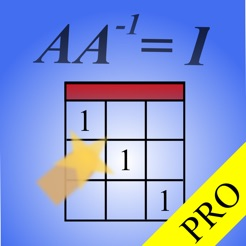## Screenshots

•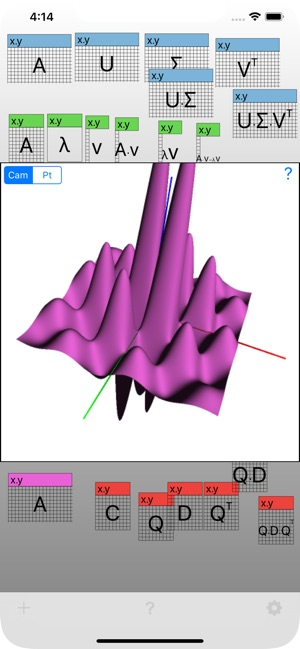•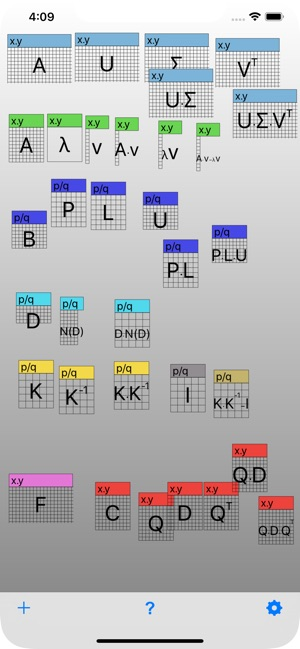•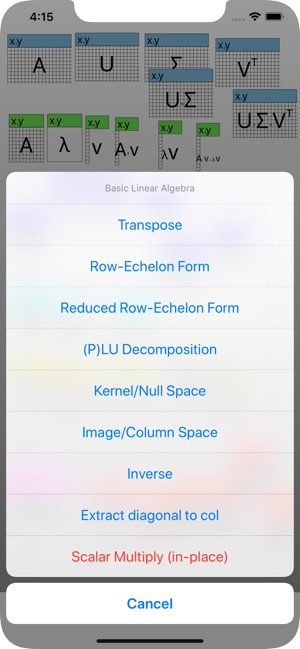•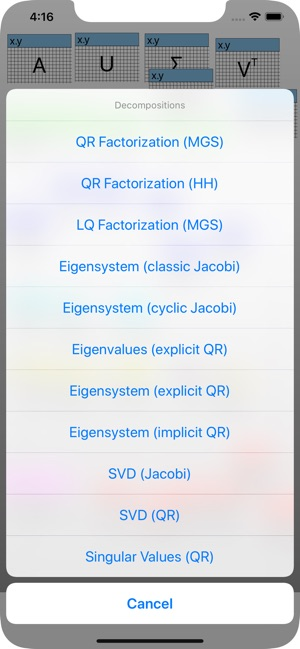•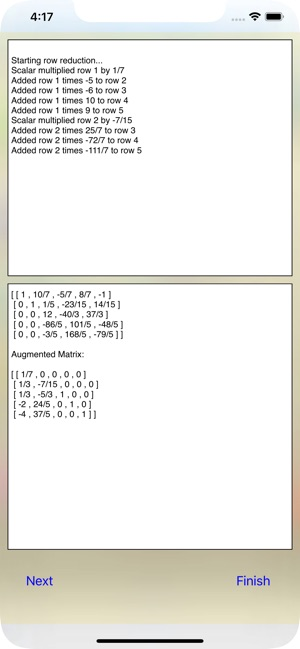•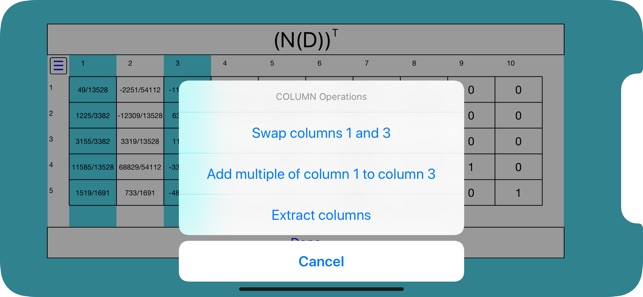•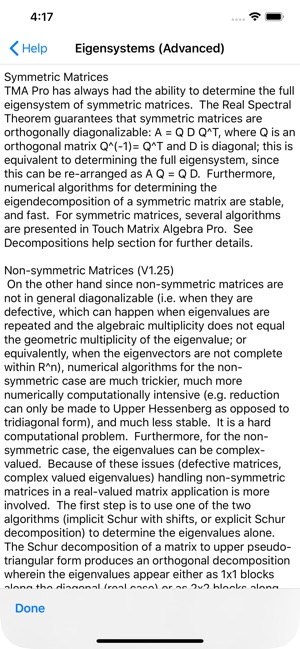•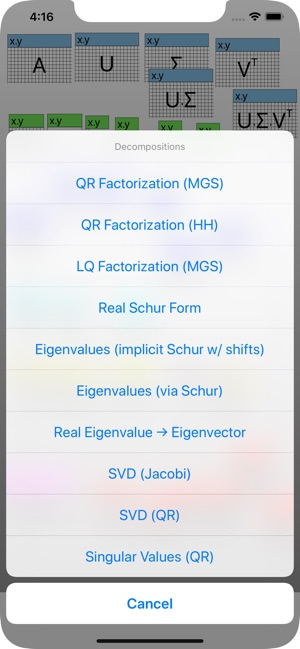## Description

Get the world’s most intuitive and advanced numerical linear algebra software and interact with numbers and matrices in a completely unique way — by touch!

Touch Matrix Algebra Professional Version not only comes with all of the in-app features of Touch Matrix Algebra unlocked — unlimited matrix sizes, 3D graphing — it adds, in addition to all the basic linear algebra operations, the most important advanced algorithms from advanced numerical linear algebra: orthogonal transforms, reductions and iterative matrix decompositions like QR factorization (declared the most important algorithm of the 20th century), symmetric eigensystem determination and singular value decomposition.

Unique features carried over from 'Touch Matrix Algebra’ include the ability to:

• drag and drop colour coded matrices to perform binary operations (e.g. matrix multiplication);
• work with either arbitrary precision matrix elements as rational numbers (rational matrices) for basic rational algorithms, or conventional fixed-precision, floating point numbers (decimal matrices) for all algorithms. Convert the former into the latter;
• see the detailed, step-by-step calculation of the Gaussian algorithm that brings a matrix to row-echelon or reduced row-echelon form, and calculates a matrix inverse, rank or determinant (for rational matrices)...
• or better yet... in editing mode, highlight rows and instruct the app to carry out the elementary row operations yourself! Learn the essence of the Gaussian algorithm without the hassle of carrying out the arithmetic details.
• factorize a matrix into Lower and Upper triangular matrices (up to a Permutation matrix): PLU decomposition.
• compute fundamental subspaces of a matrix (kernel and image)
• solve linear systems, including over and under-determined ones, via drag and drop!

The additional advanced features of Touch Matrix Algebra PRO include:

• 3D Graphing: turns Touch Matrix Algebra into a beautiful, inexpensive 3D graphing calculator that you can manipulate by panning and pinching; 3D graph analytic expressions, or visualize your large matrices' data content.
• orthogonal transforms: Householder reflections, Givens and Jacobi rotations: perfect for learning how the reductions and advanced decompositions are carried out numerically — no other software package explicitly includes these;
• matrix reductions: to bidiagonal, and tridiagonal or upper Hessenberg form (including the orthogonal matrices that carry out the reductions) — again unique to Touch Matrix Algebra Pro;
• non-iterative factorizations: QR/LQ (Modified-Gram Schmidt), QR (Householder)
• iterative decompositions: diagonalization/eigensystem determination for symmetric matrices (via explicit or implicit QR, cyclic Jacobi or a novel O(n) for large n classic Jacobi algorithm), real Schur decomposition/eigenvalue determination for non symmetric matrices, and the all-powerful singular value decomposition (via either QR or Jacobi).
• sub matrix editing, elementary column operations (in addition to the existing row operations), drag-and-drop scalar multiplication (e.g. for verifying eigenvalue-eigenvector pairs), and a Frobenius norm computation to check the results of decompositions or compare matrices.

All of the advanced algorithms have been coded lovingly by hand from scratch, are ‘duplicated’ by alternate algorithms when possible (e.g. Jacobi vs QR) and supply the transformations all of which allow you to verify the results directly. The O(n^3) algorithms have been threaded so that you can carry on several computations at once, or merely watch the iterative convergence of large matrix decompositions. Some parallelizable algorithms have themselves been multithreaded.

The Pro version now supports data import and export for communication with spreadsheets, and other numerical analysis software.

Designed by an educator, mathematician and physicist.

Coded in Swift, Objective-C, and C++.

## What’s New

Version 1.25

• Re-wrote the 3D graphing to use Metal for 3D rendering (by default) since Apple deprecated OpenGL June 2018.
• Added *non*-symmetric matrix eigenvector determination using a variation of inverse iteration (for individual eigenvalues, due to the defective matrix problem of presenting all of the eigenspaces at once), and a second implicit Schur algorithm to determine all eigenvalues (real and imaginary parts, for nonsymmetric matrices). Either drop a scalar (1x1 matrix) 'guess'/initial seed onto a square matrix, or select 'Eigenvalue->Eigenvector' to manually enter the initial eigenvalue seed. [Note: Eigendecomposition via orthogonal diagonalization for *symmetric* matrices using several algorithms was always part of TMA Pro under 'Decompositions'. If you have a feature request please let me know directly, instead of by via review.]
• added convenience features: extract diagonal as column, row/column vector to diagonal matrix
• can now manually re-color matrices via RGB sliders
• linear solver improvements, and general bug fixes.
• Updated for iOS 12 and new devices

2.0 out of 5
1 Rating

1 Rating

## Information

Seller
Kirk Kaminsky
Size
18.2 MB
Category
Education
Compatibility

Requires iOS 9.1 or later. Compatible with iPhone, iPad, and iPod touch.

Languages

English

Age Rating
Rated 4+
Copyright
© 2015-2019 Kirk Kaminsky
Price
\$4.99

## Supports

•